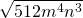# 9.1 Reducing Square Roots

Square roots are the most common type of radical. A square will take some number and multiply it by itself. A square root of a number gives the number that, when multiplied by itself, gives the number shown beneath the radical. For example, because 52 = 25, the square root of 25 is 5.

The square root of 25 is written as √25 or as 25½.

Example 9.1.1

Solve the following square roots: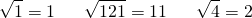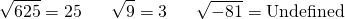The final example, √−81, is classified as being undefined in the real number system since negatives have no square root. This is because if you square a positive or a negative, the answer will be positive. This means that when using the real number system, take only square roots of positive numbers. There are solutions to negative square roots, but they require a new number system to be created that is termed the imaginary number system. For now, simply say they are undefined in the real number system or that they have no real solution

Not all numbers have a nice even square root. For example, if you look up √8 on your calculator, the answer would be 2.828427124746190097603377448419…, with this number being a rounded approximation of the square root. The standard for radicals that have large, rounded solutions is that the calculator is not used to find decimal approximations of square roots. Instead, express roots in simplest radical form.

There are a number of properties that can be used when working with radicals. One is known as the product rule: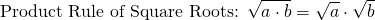Use the product rule to simplify an expression by finding perfect squares that divide evenly into the radicand (the number under the radical). Commonly used perfect squares are: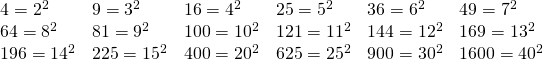The challenge in reducing radicals is often simplified to finding the perfect square to divide into the radicand.

Example 9.1.2

Find the perfect squares that divide evenly into the radicand.

1.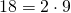2.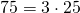3.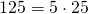4.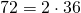5.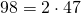6.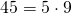Combining the strategies used in the above two examples makes the simplest strategy to reduce radicals.

Example 9.1.3

Reduce √75.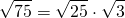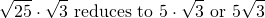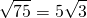If there is a coefficient in front of the radical to begin with, the problem merely becomes a big multiplication problem.

Example 9.1.4

Reduce 5√63.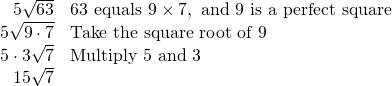Variables often are part of the radicand as well. When taking the square roots of variables, divide the exponent by 2.

For example, √x8 = x4, because you divide the exponent 8 by 2. This follows from the power of a power rule of exponents, (x4)2 = x8. When squaring, multiply the exponent by two, so when taking a square root, divide the exponent by 2. This is shown in the following example.

Example 9.1.5

Reduce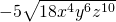.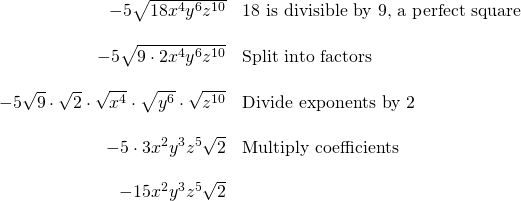Sometimes, you cannot evenly divide the exponent on a variable by 2. Sometimes, there is a remainder. If there is a remainder, this means the remainder is left inside the radical, and the whole number part goes outside the radical. This is shown in the following example.

Example 9.1.6

Reduce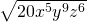.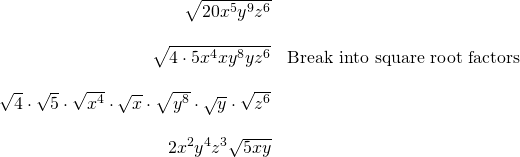Example 9.1.7

Reduce.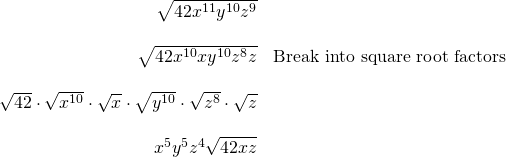# Questions

Simplify the following radicals.

1.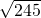2.3.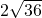4.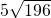5.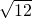6.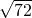7.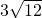8.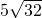9.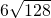10.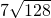11.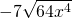12.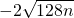13.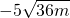14.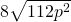15.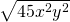16.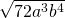17.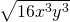18.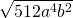19.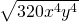20.